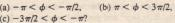# QUESTIONS

QUESTIONS

1. Which of the following relationships between the acceleration a and the displacement x of a panicle involve SHM: (a) a = 0.5x, (b) a = 400×2, (c) a = -2Ox, (d) a = -3×2? 2. Given x’ =·(2.0 m) cos(5t) for SHM and needing to find the velocity at t = 2 s, should you substitute for t and then differentiate with respect to t or vice versa  The acceleration a(t) of a particle undergoing SHM is graphed in (a) Which of the labeled points corresponds to the panicle at – m?  b) At point 4, is the velocity of the particle positive negative, or zero? (c) At 6 point 5, is the panicle at at +xm’ at 0, between -Xm and 0 Question  or between 0 and  4. Which of the following describe <I> for the SHMThe velocity v(t) of a panicle undergoing SHM is graphed in
Fig. 16-19b. I~ the panicle momentarily stajionary, headed toward
-xm• or headed toward +xm at (a) point A on, the graph and
(b) pointB? Is the panicle at -Xm• at +x.,. at 0, between -xm and
0, or ‘between 0 and +xm when its velocity is represented by
(c) point A and (d) point B? Is the speed df the panicle increasing
or decreasing at (e) point A and (I) point B?